# 5. LDA 文本建模

## 5.1 游戏规则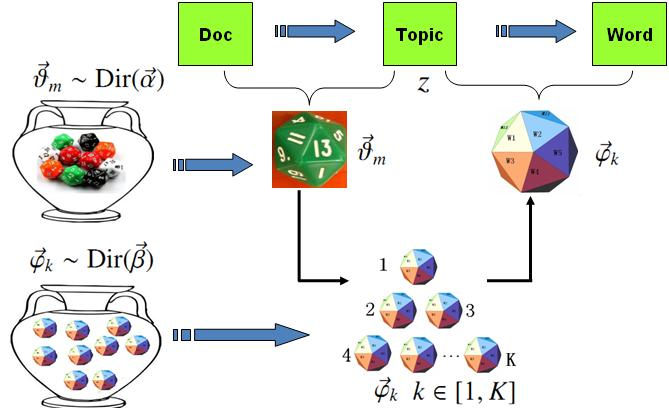LDA模型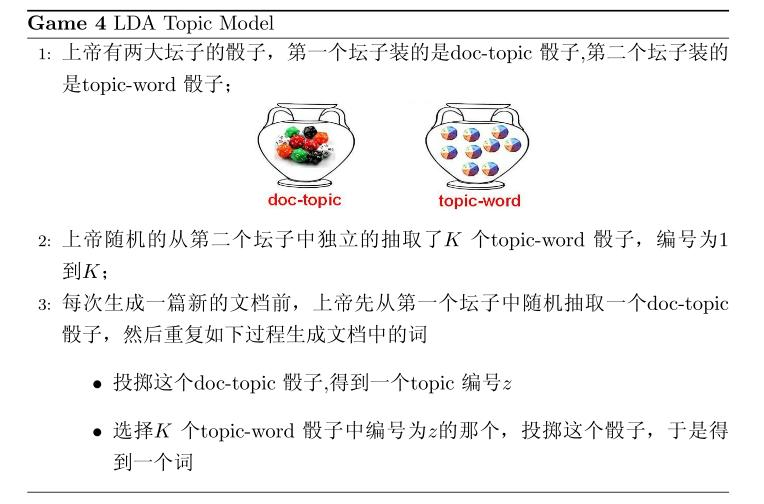\begin{align*} \overrightarrow{\mathbf{w}} & = (\overrightarrow{w}_1, \cdots, \overrightarrow{w}_M) \\ \overrightarrow{\mathbf{z}} & = (\overrightarrow{z}_1, \cdots, \overrightarrow{z}_M) \end{align*}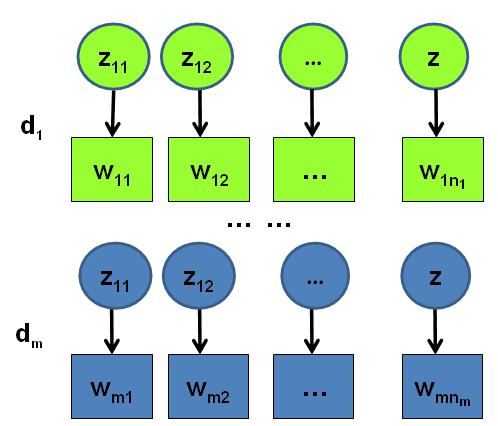## 5.2 物理过程分解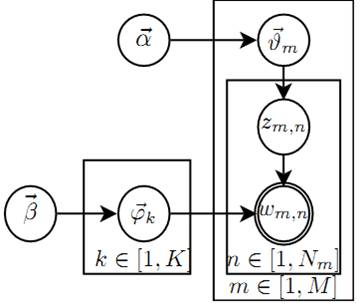LDA概率图模型表示

•  $\overrightarrow{\alpha}\rightarrow \overrightarrow{\theta}_m \rightarrow z_{m,n}$， 这个过程表示在生成第$m$ 篇文档的时候，先从第一个坛子中抽了一个doc-topic 骰子$\overrightarrow{\theta}_m$， 然后投掷这个骰子生成了文档中第$n$ 个词的topic编号$z_{m,n}$
•  $\overrightarrow{\beta} \rightarrow \overrightarrow{\varphi}_k \rightarrow w_{m,n} | k=z_{m,n}$， 这个过程表示用如下动作生成语料中第$m$篇文档的第$n$个词：在上帝手头的$K$个topic-word 骰子 $\overrightarrow{\varphi}_k$中，挑选编号为$k=z_{m,n}$的那个骰子进行投掷，然后生成 word$w_{m,n}$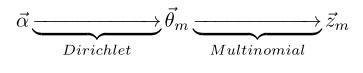$$p(\overrightarrow{z}_m |\overrightarrow{\alpha}) = \frac{\Delta(\overrightarrow{n}_m+\overrightarrow{\alpha})}{\Delta(\overrightarrow{\alpha})}$$

$$Dir(\overrightarrow{\theta}_m| \overrightarrow{n}_m + \overrightarrow{\alpha}).$$

\begin{align} \label{corpus-topic-prob} p(\overrightarrow{\mathbf{z}} |\overrightarrow{\alpha}) & = \prod_{m=1}^M p(\overrightarrow{z}_m |\overrightarrow{\alpha}) \notag \\ &= \prod_{m=1}^M \frac{\Delta(\overrightarrow{n}_m+\overrightarrow{\alpha})}{\Delta(\overrightarrow{\alpha})} \quad\quad (*) \end{align}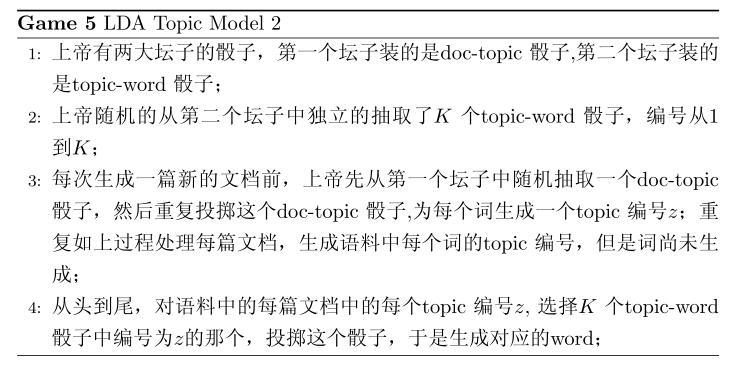\begin{align*} \overrightarrow{\mathbf{w}}’ &= (\overrightarrow{w}_{(1)}, \cdots, \overrightarrow{w}_{(K)}) \\ \overrightarrow{\mathbf{z}}’ &= (\overrightarrow{z}_{(1)}, \cdots, \overrightarrow{z}_{(K)}) \end{align*}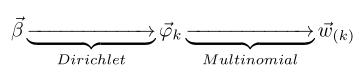$$p(\overrightarrow{w}_{(k)} |\overrightarrow{\beta}) = \frac{\Delta(\overrightarrow{n}_k+\overrightarrow{\beta})}{\Delta(\overrightarrow{\beta})}$$

$$Dir( \overrightarrow{\varphi}_k| \overrightarrow{n}_k + \overrightarrow{\beta}).$$

\begin{align} \label{corpus-word-prob} p(\overrightarrow{\mathbf{w}} |\overrightarrow{\mathbf{z}},\overrightarrow{\beta}) &= p(\overrightarrow{\mathbf{w}}’ |\overrightarrow{\mathbf{z}}’,\overrightarrow{\beta}) \notag \\ &= \prod_{k=1}^K p(\overrightarrow{w}_{(k)} | \overrightarrow{z}_{(k)}, \overrightarrow{\beta}) \notag \\ &= \prod_{k=1}^K \frac{\Delta(\overrightarrow{n}_k+\overrightarrow{\beta})}{\Delta(\overrightarrow{\beta})}  \quad\quad (**) \end{align}

\begin{align} \label{lda-corpus-likelihood} p(\overrightarrow{\mathbf{w}},\overrightarrow{\mathbf{z}} |\overrightarrow{\alpha}, \overrightarrow{\beta}) &= p(\overrightarrow{\mathbf{w}} |\overrightarrow{\mathbf{z}}, \overrightarrow{\beta}) p(\overrightarrow{\mathbf{z}} |\overrightarrow{\alpha}) \notag \\ &= \prod_{k=1}^K \frac{\Delta(\overrightarrow{n}_k+\overrightarrow{\beta})}{\Delta(\overrightarrow{\beta})} \prod_{m=1}^M \frac{\Delta(\overrightarrow{n}_m+\overrightarrow{\alpha})}{\Delta(\overrightarrow{\alpha})}   (***) \end{align}

## 5.3 Gibbs Sampling

\begin{align*} p(z_i = k|\overrightarrow{\mathbf{z}}_{\neg i}, \overrightarrow{\mathbf{w}}) \propto p(z_i = k, w_i = t |\overrightarrow{\mathbf{z}}_{\neg i}, \overrightarrow{\mathbf{w}}_{\neg i}) \\ \end{align*}

1. $\overrightarrow{\alpha} \rightarrow \overrightarrow{\theta}_m \rightarrow \overrightarrow{z}_{m}$
2. $\overrightarrow{\beta} \rightarrow \overrightarrow{\varphi}_k \rightarrow \overrightarrow{w}_{(k)}$

\begin{align*} p(\overrightarrow{\theta}_m|\overrightarrow{\mathbf{z}}_{\neg i}, \overrightarrow{\mathbf{w}}_{\neg i}) &= Dir(\overrightarrow{\theta}_m| \overrightarrow{n}_{m,\neg i} + \overrightarrow{\alpha}) \\ p(\overrightarrow{\varphi}_k|\overrightarrow{\mathbf{z}}_{\neg i}, \overrightarrow{\mathbf{w}}_{\neg i}) &= Dir( \overrightarrow{\varphi}_k| \overrightarrow{n}_{k，\neg i} + \overrightarrow{\beta}) \end{align*}

\begin{align*} p(z_i = k|\overrightarrow{\mathbf{z}}_{\neg i}, \overrightarrow{\mathbf{w}}) & \propto p(z_i = k, w_i = t |\overrightarrow{\mathbf{z}}_{\neg i}, \overrightarrow{\mathbf{w}}_{\neg i}) \\ &= \int p(z_i = k, w_i = t, \overrightarrow{\theta}_m,\overrightarrow{\varphi}_k | \overrightarrow{\mathbf{z}}_{\neg i}, \overrightarrow{\mathbf{w}}_{\neg i}) d \overrightarrow{\theta}_m d \overrightarrow{\varphi}_k \\ &= \int p(z_i = k, \overrightarrow{\theta}_m|\overrightarrow{\mathbf{z}}_{\neg i}, \overrightarrow{\mathbf{w}}_{\neg i}) \cdot p(w_i = t, \overrightarrow{\varphi}_k | \overrightarrow{\mathbf{z}}_{\neg i}, \overrightarrow{\mathbf{w}}_{\neg i}) d \overrightarrow{\theta}_m d \overrightarrow{\varphi}_k \\ &= \int p(z_i = k |\overrightarrow{\theta}_m) p(\overrightarrow{\theta}_m|\overrightarrow{\mathbf{z}}_{\neg i}, \overrightarrow{\mathbf{w}}_{\neg i}) \cdot p(w_i = t |\overrightarrow{\varphi}_k) p(\overrightarrow{\varphi}_k|\overrightarrow{\mathbf{z}}_{\neg i}, \overrightarrow{\mathbf{w}}_{\neg i}) d \overrightarrow{\theta}_m d \overrightarrow{\varphi}_k \\ &= \int p(z_i = k |\overrightarrow{\theta}_m) Dir(\overrightarrow{\theta}_m| \overrightarrow{n}_{m,\neg i} + \overrightarrow{\alpha}) d \overrightarrow{\theta}_m \\ & \hspace{0.2cm} \cdot \int p(w_i = t |\overrightarrow{\varphi}_k) Dir( \overrightarrow{\varphi}_k| \overrightarrow{n}_{k,\neg i} + \overrightarrow{\beta}) d \overrightarrow{\varphi}_k \\ &= \int \theta_{mk} Dir(\overrightarrow{\theta}_m| \overrightarrow{n}_{m,\neg i} + \overrightarrow{\alpha}) d \overrightarrow{\theta}_m \cdot \int \varphi_{kt} Dir( \overrightarrow{\varphi}_k| \overrightarrow{n}_{k,\neg i} + \overrightarrow{\beta}) d \overrightarrow{\varphi}_k \\ &= E(\theta_{mk}) \cdot E(\varphi_{kt}) \\ &= \hat{\theta}_{mk} \cdot \hat{\varphi}_{kt} \\ \label{gibbs-sampling-deduction} \end{align*}

\begin{align*} \hat{\theta}_{mk} &= \frac{n_{m,\neg i}^{(k)} + \alpha_k}{\sum_{k=1}^K (n_{m,\neg i}^{(k)} + \alpha_k)} \\ \hat{\varphi}_{kt} &= \frac{n_{k,\neg i}^{(t)} + \beta_t}{\sum_{t=1}^V (n_{k,\neg i}^{(t)} + \beta_t)} \end{align*}

\begin{equation} \label{gibbs-sampling} p(z_i = k|\overrightarrow{\mathbf{z}}_{\neg i}, \overrightarrow{\mathbf{w}}) \propto \frac{n_{m,\neg i}^{(k)} + \alpha_k}{\sum_{k=1}^K (n_{m,\neg i}^{(k)} + \alpha_k)} \cdot \frac{n_{k,\neg i}^{(t)} + \beta_t}{\sum_{t=1}^V (n_{k,\neg i}^{(t)} + \beta_t)} \end{equation}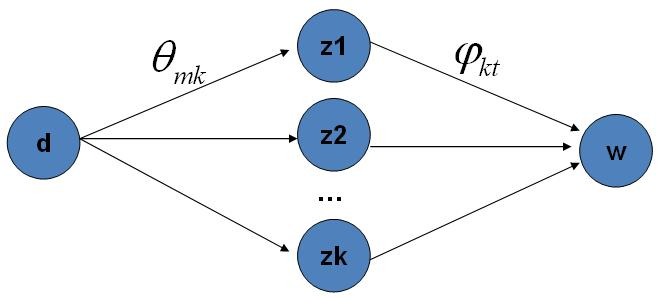doc-topic-word 路径概率

## 5.4 Training and Inference

• 估计模型中的参数$\overrightarrow{\varphi}_1, \cdots, \overrightarrow{\varphi}_K$$\overrightarrow{\theta}_1, \cdots, \overrightarrow{\theta}_M$
• 对于新来的一篇文档$doc_{new}$，我们能够计算这篇文档的 topic 分布$\overrightarrow{\theta}_{new}$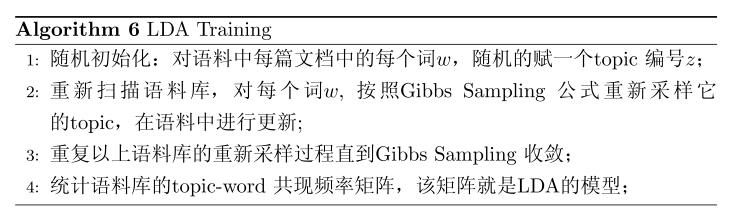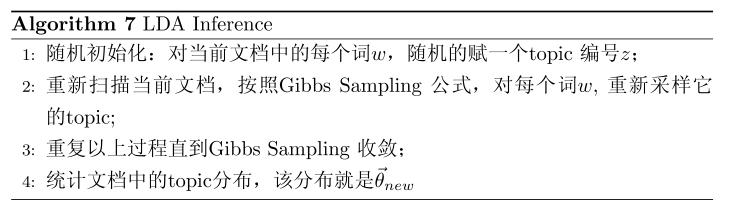# 6. 后记

LDA 对于专业做机器学习的兄弟而言，只能算是一个简单的Topic Model。但是对于互联网中做数据挖掘、语义分析的工程师，LDA 的门槛并不低。 LDA 典型的属于这样一种机器学习模型：要想理解它，需要比较多的数学背景，要在工程上进行实现，却相对简单。 Gregor Heinrich 的LDA 模型科普文章 Parameter estimation for text analysis 写得非常的出色，这是学习 LDA 的必看文章。不过即便是这篇文章，对于工程师也是有门槛的。我写的这个科普最好对照 Gregor Heinrich 的这篇文章来看， 我用的数学符号也是尽可能和这篇文章保持一致。

What I cannot create, I do not understand.

— Richard Feynman

# LDA数学八卦

LDA-math 的汇总， “LDA数学八卦.pdf” 我整理贴出来了， 希望对大家理解 LDA 有帮助。 文章标题挂上“八卦”两字， 因为八卦意味着形式自由、不拘束、可以天马行空，细节处理上也难免有不严谨的地方；当然我也希望八卦是相对容易理解的。

LDA数学八卦.pdf 下载